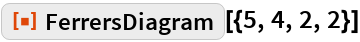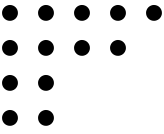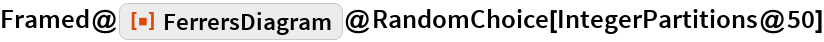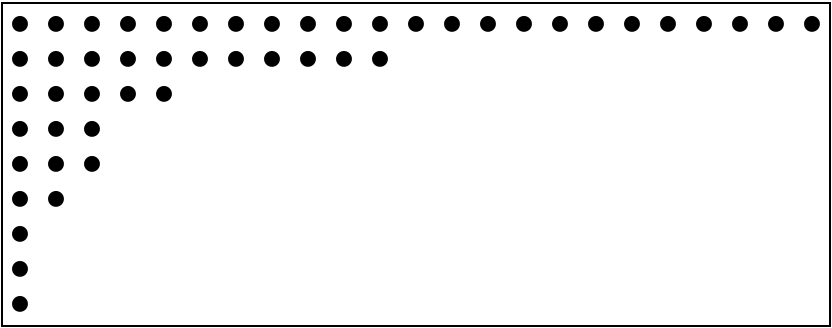#Function Repository Resource:

# FerrersDiagram

Display the Ferrers diagram of a partition with dots

Contributed by: Wolfram Staff
 ResourceFunction["FerrersDiagram"][p] gives a ragged array of dots, with the number of dots in each row equal to a corresponding part of the integer partition p.

## Details and Options

An integer partition of a positive integer n is a weakly decreasing list of positive integers that sum to n.

## Examples

### Basic Examples

Rows 1 to 4 have 5, 4, 2, 2 dots:

 In:=Out=Here is a random choice of one of the 204226 partitions of 50:

 In:=Out=